Electric Circuits - Lesson 2 - Electric Current

# Electric CurrentIf the two requirements of an electric circuit are met, then charge will flow through the external circuit. It is said that there is a current - a flow of charge. Using the word current in this context is to simply use it to say that something is happening in the wires - charge is moving. Yet current is a physical quantity that can be measured and expressed numerically. As a physical quantity, current is the rate at which charge flows past a point on a circuit. As depicted in the diagram below, the current in a circuit can be determined if the quantity of charge Q passing through a cross section of a wire in a time t can be measured. The current is simply the ratio of the quantity of charge and time.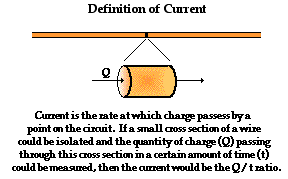Current is a rate quantity. There are several rate quantities in physics. For instance, velocity is a rate quantity - the rate at which an object changes its position. Mathematically, velocity is the position change per time ratio. Acceleration is a rate quantity - the rate at which an object changes its velocity. Mathematically, acceleration is the velocity change per time ratio. And power is a rate quantity - the rate at which work is done on an object. Mathematically, power is the work per time ratio. In every case of a rate quantity, the mathematical equation involves some quantity over time. Thus, current as a rate quantity would be expressed mathematically as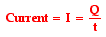Note that the equation above uses the symbol I to represent the quantity current.

As is the usual case, when a quantity is introduced in The Physics Classroom, the standard metric unit used to express that quantity is introduced as well. The standard metric unit for current is the ampere. Ampere is often shortened to Amp and is abbreviated by the unit symbol A. A current of 1 ampere means that there is 1 coulomb of charge passing through a cross section of a wire every 1 second.

1 ampere = 1 coulomb / 1 second

To test your understanding, determine the current for the following two situations. Note that some extraneous information is given in each situation. Click the Check Answer button to see if you are correct.

A 2 mm long cross section of wire is isolated and 20 C of charge is determined to pass through it in 40 s.A 1 mm long cross section of wire is isolated and 2 C of charge is determined to pass through it in 0.5 s.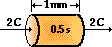I = _____ Ampere

I = _____ Ampere

### Conventional Current Direction

The particles that carry charge through wires in a circuit are mobile electrons. The electric field direction within a circuit is by definition the direction that positive test charges are pushed. Thus, these negatively charged electrons move in the direction opposite the electric field. But while electrons are the charge carriers in metal wires, the charge carriers in other circuits can be positive charges, negative charges or both. In fact, the charge carriers in semiconductors, street lamps and fluorescent lamps are simultaneously both positive and negative charges traveling in opposite directions.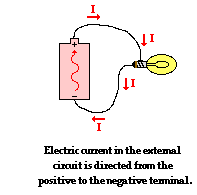Ben Franklin, who conducted extensive scientific studies in both static and current electricity, envisioned positive charges as the carriers of charge. As such, an early convention for the direction of an electric current was established to be in the direction that positive charges would move. The convention has stuck and is still used today. The direction of an electric current is by convention the direction in which a positive charge would move. Thus, the current in the external circuit is directed away from the positive terminal and toward the negative terminal of the battery. Electrons would actually move through the wires in the opposite direction. Knowing that the actual charge carriers in wires are negatively charged electrons may make this convention seem a bit odd and outdated. Nonetheless, it is the convention that is used worldwide and one that a student of physics can easily become accustomed to.

### Current versus Drift Speed

Current has to do with the number of coulombs of charge that pass a point in the circuit per unit of time. Because of its definition, it is often confused with the quantity drift speed. Drift speed refers to the average distance traveled by a charge carrier per unit of time. Like the speed of any object, the drift speed of an electron moving through a wire is the distance to time ratio.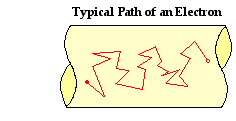The path of a typical electron through a wire could be described as a rather chaotic, zigzag path characterized by collisions with fixed atoms. Each collision results in a change in direction of the electron. Yet because of collisions with atoms in the solid network of the metal conductor, there are two steps backwards for every three steps forward. With an electric potential established across the two ends of the circuit, the electron continues to migrate forward. Progress is always made towards the positive terminal. Yet the overall effect of the countless collisions and the high between-collision speeds is that the overall drift speed of an electron in a circuit is abnormally low. A typical drift speed might be 1 meter per hour. That is slow!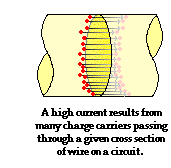One might then ask: How can there by a current on the order of 1 or 2 ampere in a circuit if the drift speed is only about 1 meter per hour? The answer is: there are many, many charge carriers moving at once throughout the whole length of the circuit. Current is the rate at which charge crosses a point on a circuit. A high current is the result of several coulombs of charge crossing over a cross section of a wire on a circuit. If the charge carriers are densely packed into the wire, then there does not have to be a high speed to have a high current. That is, the charge carriers do not have to travel a long distance in a second, there just has to be a lot of them passing through the cross section. Current does not have to do with how far charges move in a second but rather with how many charges pass through a cross section of wire on a circuit.

To illustrate how densely packed the charge carriers are, we will consider a typical wire found in household lighting circuits - a 14-gauge copper wire. In a 0.01 cm-long (very thin) cross-sectional slice of this wire, there would be as many as 3.51 x 1020 copper atoms. Each copper atom has 29 electrons; it would be unlikely that even the 11 valence electrons would be in motion as charge carriers at once. If we assume that each copper atom contributes just a single electron, then there would be as much as 56 coulombs of charge within a thin 0.01-cm length of the wire. With that much mobile charge within such a small space, a small drift speed could lead to a very large current.

To further illustrate this distinction between drift speed and current, consider this racing analogy. Suppose that there was a very large turtle race with millions and millions of turtles on a very wide race track. Turtles do not move very fast - they have a very low drift speed. Suppose that the race was rather short - say 1 meter in length - and that a large percentage of the turtles reached the finish line at the same time - 30 minutes after the start of the race. In such a case, the current would be very large - with millions of turtles passing a point in a short amount of time. In this analogy, speed has to do with how far the turtles move in a certain amount of time; and current has to do with how many turtles cross the finish line in a certain amount of time.

### The Nature of Charge Flow

Once it has been established that the average drift speed of an electron is very, very slow, the question soon arises: Why does the light in a room or in a flashlight light immediately after the switched is turned on? Wouldn't there be a noticeable time delay before a charge carrier moves from the switch to the light bulb filament? The answer is NO! and the explanation of why reveals a significant amount about the nature of charge flow in a circuit.

As mentioned above, charge carriers in the wires of electric circuits are electrons. These electrons are simply supplied by the atoms of copper (or whatever material the wire is made of) within the metal wire. Once the switch is turned to on, the circuit is closed and there is an electric potential difference is established across the two ends of the external circuit. The electric field signal travels at nearly the speed of light to all mobileelectrons within the circuit, ordering them to begin marching. As the signal is received, the electrons begin moving along a zigzag path in their usual direction. Thus, the flipping of the switch causes an immediate response throughout every part of the circuit, setting charge carriers everywhere in motion in the same net direction. While the actual motion of charge carriers occurs with a slow speed, the signal that informs them to start moving travels at a fraction of the speed of light.

The electrons that light the bulb in a flashlight do not have to first travel from the switch through 10 cm of wire to the filament. Rather, the electrons that light the bulb immediately after the switch is turned to on are the electrons that are present in the filament itself. As the switch is flipped, all mobile electrons everywhere begin marching; and it is the mobile electrons present in the filament whose motion are immediately responsible for the lighting of its bulb. As those electrons leave the filament, new electrons enter and become the ones that are responsible for lighting the bulb. The electrons are moving together much like the water in the pipes of a home move. When a faucet is turned on, it is the water in the faucet that emerges from the spigot. One does not have to wait a noticeable time for water from the entry point to your home to travel through the pipes to the spigot. The pipes are already filled with water and water everywhere within the water circuit is set in motion at the same time.

The picture of charge flow being developed here is a picture in which charge carriers are like soldiers marching along together, everywhere at the same rate. Their marching begins immediately in response to the establishment of an electric potential across the two ends of the circuit. There is no place in the electrical circuitwhere charge carriers become consumed or used up. While the energy possessed by the charge may be used up (or a better way of putting this is to say that the electric energy is transformed to other forms of energy), the charge carriers themselves do not disintegrate, disappear or otherwise become removed from the circuit. And there is no place in the circuit where charge carriers begin to pile up or accumulate. The rate at which charge enters the external circuit on one end is the same as the rate at which charge exits the external circuit on the other end. Current - the rate of charge flow - is everywhere the same. Charge flow is like the movement of soldiers marching in step together, everywhere at the same rate.

1. A current is said to exist whenever _____.

a. a wire is charged

b. a battery is present

c. electric charges are unbalanced

d. electric charges move in a loop

2. Current has a direction. By convention, current is in the direction that ___.

a. + charges move

b. - electrons move

c. + electrons move

3. The drift velocity of mobile charge carriers in electric circuits is ____.

a. very fast; less than but very close to the speed of light

b. fast; faster than the fastest car but nowhere near the speed of light

c. slow; slower than Michael Jackson runs the 220-meters

d. very slow; slower than a snail

4. If an electric circuit could be compared to a water circuit at a water park, then the current would be analogous to the ____.

Choices:

 A. water pressure B. gallons of water flowing down slide per minute C. water D. bottom of the slide E. water pump F. top of the slide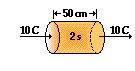5. The diagram at the right depicts a conducting wire. Two cross-sectional areas are located 50 cm apart. Every 2.0 seconds, 10 C of charge flow through each of these areas. The current in this wire is ____ A.

 a. 0.10 b. 0.25 c. 0.50 d. 1.0 e. 5.0 f. 20 g. 10 h. 40 i. none of these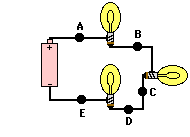6. Use the diagram at the right to complete the following statements:

a. A current of one ampere is a flow of charge at the rate of _______ coulomb per second.

b. When a charge of 8 C flows past any point along a circuit in 2 seconds, the current is ________ A.

c. If 5 C of charge flow past point A (diagram at right) in 10 seconds, then the current is _________ A.

d. If the current at point D is 2.0 A, then _______ C of charge flow past point D in 10 seconds.

e. If 12 C of charge flow past point A in 3 seconds, then 8 C of charge will flow past point E in ________ seconds.

f. True or False:

The current at point E is considerably less than the current at point A since charge is being used up in the light bulbs.

Next Section: